International
Tables for
Crystallography
Volume B
Reciprocal space
Edited by U. Shmueli

International Tables for Crystallography (2006). Vol. B. ch. 4.5, pp. 471-472   | 1 | 2 |

## Section 4.5.2.4.4. Partially crystalline fibres

R. P. Millanea*

#### 4.5.2.4.4. Partially crystalline fibres

| top | pdf |

In this section we address disorder in the packing of the molecules in a polycrystalline fibre. The presence of disorder within the crystallites modifies the intensities of the Bragg reflections, as well as introducing continuous diffraction. The dominant effect, sometimes seen on fibre diffraction patterns (Stroud & Millane, 1995a), is for Bragg reflections to remain at low resolution but to be replaced by continuous diffraction at high resolution. There are two distinct cases to consider. The first is where the distortions at different lattice points in the crystallite are uncorrelated, and the second is where they are correlated.

Disorder within a crystallite in a polycrystalline fibre may consist of (1) deviations in the positions of the molecules (which are treated as rigid bodies) from their positions in a regular lattice, (2) rotations of the molecules about their molecular axes from their rotational positions in an ordered crystal, and (3) random orientations (up or down) of the molecules. The first of these is called lattice disorder, and the second and third are components of substitution disorder.

Uncorrelated disorder has been treated by a number of authors (Clark & Muus, 1962; Tanaka & Naya, 1969; Fraser & MacRae, 1973; Arnott, 1980). A rather complete model has been described by Millane & Stroud (1991)and Stroud & Millane (1995b), which is presented here. If the lattice and substitution disorders are independent, and the lattice and substitution distortions at different lattice sites are uncorrelated, then the cylindrically averaged layer-line intensities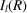diffracted from a fibre can be written as a sum of Bragg and diffuse (continuous) intensities (Tanaka & Naya, 1969):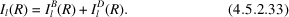The profiles of the Bragg reflections are independent of the position of the reflection in reciprocal space. If the Cartesian components of the lattice distortions are independent, normally distributed, and the x and y components have equal variances, cylindrical averaging of the diffracted intensity can be performed analytically.

The lattice disorder consists of distortions of the two-dimensional lattice (in the lateral plane) into three-dimensional space, and in the absence of substitution disorder the Bragg component is given by (Stroud & Millane, 1995b)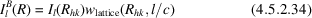where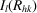is given by equation (4.5.2.24), the lattice disorder weight,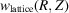, is given by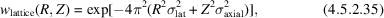where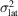and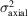are the variances of the lattice distortions normal (lateral') and parallel (axial') to the z axis, respectively. The diffuse component is given by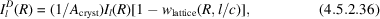whereis given by equation (4.5.2.17)and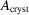is the average cross-sectional area of the crystallites. Inspection of equations (4.5.2.34)and (4.5.2.36)shows that the effect of the lattice disorder is to weight the amplitudes of the Bragg reflections down with increasing R and l, and to introduce a continuous intensity component whose amplitude increases with R and Z. Furthermore, the amplitude of the diffuse component relative to the Bragg component is inversely proportional to, and therefore is not significant unless the crystallites are small.

If substitution disorder is also present, then equation (4.5.2.33)still applies but equations (4.5.2.34)and (4.5.2.36)are replaced by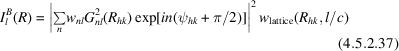and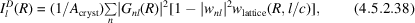respectively, where the substitution disorder weight,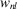, is given by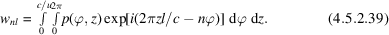In equation (4.5.2.39),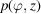is the probability density function (p.d.f.) that describes the substitution disorder, i.e. the p.d.f. for a molecule being rotated by ϕ about and translated by z along the molecular axis relative to its position in the undistorted lattice. Inspection of equations (4.5.2.37)and (4.5.2.38)shows that the substitution disorder weights the different contributing Bessel terms differently. This can lead to quite complicated effects on the diffraction pattern for various kinds of substitution disorder, resulting in different distributions and amplitudes of Bragg and diffuse diffraction over the diffraction pattern (Stroud & Millane, 1995b). If one assumes either uniform or normal distributions for ϕ and z, then expressions can be obtained for thein terms of the variances of the distributions of ϕ and z (Stroud & Millane, 1995b). The cases where distortions in ϕ are correlated with distortions in z (e.g. `screw disorder'), and directional (up and down) disorder, can also be accommodated. This model has been shown to be capable of predicting diffraction patterns which are in good agreement with those measured from some disordered polycrystalline fibres (Stroud & Millane, 1995a).

We consider now the case of correlated packing disorder. As a result of intermolecular contacts within a polycrystalline specimen, it is possible that distortions at one lattice site will affect the degree of distortion at neighbouring sites. Coupling between distortions at different lattice sites can be included in the model of disorder by allowing the distortions at different lattice sites to be correlated. The effect of correlated distortions on diffraction patterns is that the diffracted intensity does not separate into Bragg and diffuse components as it does in the case of uncorrelated distortions [equation (4.5.2.33)]. The intensity can be described as being diffuse on the whole diffraction pattern, with (often broad) maxima occurring at some of the reciprocal-lattice points, but with no significant maxima at other reciprocal-lattice points. The widths of the profiles of the maxima generally increase with increasing resolution, whereas the widths of the Bragg maxima resulting from uncorrelated disorder as described above are independent of resolution. A broadening of diffraction maxima with increasing resolution and blending into continuous diffraction is sometimes seen on diffraction patterns from polycrystalline fibres, indicating the presence of correlated disorder (Stroud & Millane, 1996b).

Correlated lattice disorder consists of correlated distortions of the two-dimensional lattice into three-dimensional space. A flexible model of crystalline disorder is that based on the perturbed lattice approach (Welberry et al., 1980). While formulating perturbed lattices with only nearest-neighbour interactions is complicated, a more tractable approach is to base the statistics on an imposed correlation field (de Graaf, 1989; Stroud & Millane, 1996a). This approach has been used to describe cylindrically averaged diffraction from polycrystalline fibres that contain correlated lattice disorder and uncorrelated substitution disorder (Stroud & Millane, 1996a,b), and is presented here.

To develop a flexible and tractable theory for diffraction from crystallites with correlated disorder, it is necessary to formulate the problem in real space. The size and shape of a crystallite in the xy (lateral) plane is described by a shape function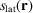, where r denotes the position vector in real space, which is equal to unity inside the crystallite and zero outside. The autocorrelation of the shape function,, is given by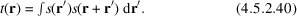The correlations between the x components, and between the y components, of the distortions at any two lattice sites are taken to be identical. The correlations between distortion vectors are defined in terms of lateral,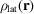, and axial,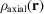, correlation fields such that the correlation coefficients between components of the distortions in the x (or y) and z directions, respectively, are equal to the correlation field evaluated for r equal to the inter-site vector. Various functional forms for the correlation fields are possible, but exponential correlation functions are usually used (Stroud & Millane, 1996a). Ifand the correlation fields are circularly symmetric, then cylindrical averaging of the diffracted intensity can be performed analytically.

For a polycrystalline fibre with correlated lattice disorder and uncorrelated substitution disorder, the diffracted intensity is given by (Stroud & Millane, 1996b)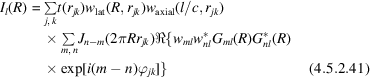where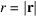, the sum over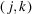is over all the sites of the undistorted lattice within the region occupied by the autocorrelation function,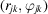are the polar coordinates of the lattice sites, and the lateral and axial lattice disorder weights are given by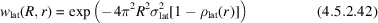and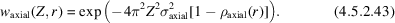Equation (4.5.2.41)is an expression for the continuous intensity distribution along the layer lines and does not separate into Bragg and continuous components as in the case of uncorrelated disorder. However, calculations using these expressions show that the continuous intensity is sharply peaked around the projected reciprocal-lattice points at low resolution, the peaks broadening with increasing resolution until they have the character of continuous diffraction at high resolution (Stroud & Millane, 1996a). This is consistent with the character of diffraction patterns from some disordered polycrystalline fibres. A detailed study of the effects of correlated disorder on fibre diffraction patterns, and analysis of such disorder, can be found in Stroud & Millane (1996a)and Stroud & Millane (1996b).

### ReferencesArnott, S. (1980). Twenty years hard labor as a fibre diffractionist. In Fibre diffraction methods, ACS Symposium Series, Vol. 141, edited by A. D. French & K. H. Gardner, pp. 1–30. Washington DC: American Chemical Society.Google ScholarClark, E. S. & Muus, I. T. (1962). The relationship between Bragg reflections and disorder in crystalline polymers. Z. Kristallogr. 117, 108–118.Google ScholarFraser, R. D. B. & MacRae, T. P. (1973). Conformations in fibrous proteins. New York: Academic Press.Google ScholarGraaf, H. de (1989). On the calculation of small-angle diffraction patterns from distorted lattices. Acta Cryst. A45, 861–870.Google ScholarMillane, R. P. & Stroud, W. J. (1991). Effects of disorder on fibre diffraction patterns. Int. J. Biol. Macromol. 13, 202–208.Google ScholarStroud, W. J. & Millane, R. P. (1995a). Analysis of disorder in biopolymer fibres. Acta Cryst. A51, 790–800.Google ScholarStroud, W. J. & Millane, R. P. (1995b). Diffraction by disordered polycrystalline fibres. Acta Cryst. A51, 771–790.Google ScholarStroud, W. J. & Millane, R. P. (1996a). Cylindrically averaged diffraction by distorted lattices. Proc. R. Soc. London, 452, 151–173.Google ScholarStroud, W. J. & Millane, R. P. (1996b). Diffraction by polycrystalline fibres with correlated disorder. Acta Cryst. A52, 812–829.Google ScholarTanaka, S. & Naya, S. (1969). Theory of X-ray scattering by disordered polymer crystals. J. Phys. Soc. Jpn, 26, 982–993.Google ScholarWelberry, T. R., Miller, G. H. & Carroll, C. E. (1980). Paracrystals and growth-disorder models. Acta Cryst. A36, 921–929.Google Scholar